# Delete a Row in VBA

We will introduce how we can delete rows for different situations with examples in VBA.

## Delete a Row in VBA

Deleting a row from an Excel sheet is an easy job that can be done using the keyboard shortcut method or a mouse or touchpad. But when we are specific about deleting a row or many rows, the VBA route is helpful to work with.

VBA is used to delete row(s) or column(s) of an Excel sheet if unnecessary fields are a part of the data. It can help the user to delete a column on a certain condition.

For example, duplicate rows can be deleted easily using the VBA command.

This article covers some of the main examples and related VBA codes that can be helpful for the user to work on Excel worksheets. These multiple scenarios include:

1. To delete an Entire Row

1.1. To delete First Row

1.2. To delete Specific Row

1. To delete Multiple Rows
2. To delete Alternative Rows
3. To delete Blank Rows
4. To delete Rows with Specific Date Values
5. To delete Duplicate Rows

## How to Delete an Entire Row in VBA

Now, let’s discuss our first scenario in which we will try to delete the first row in VBA; if we want to delete the first row, VBA’s `EntireRow.Delete` method can help us.

Now let’s use this method in our example to delete the first row of our Excel sheet.

We will create a `sub` as `deleteFirstRow()`. The code for this purpose is shown below.

``````# VBA
Sub DeleteFirstRow()
ThisWorkbook.Worksheets(1).Rows(1).EntireRow.Delete
End Sub
``````

Output: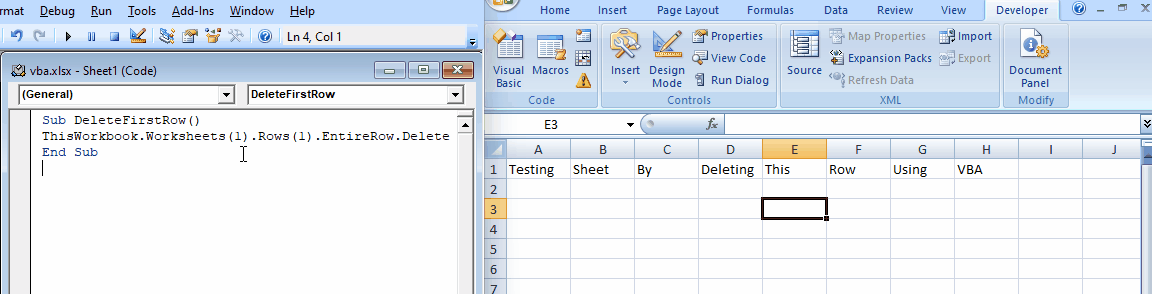The above example shows that this code works by specifying the row refers to a specific number and then uses the `EntireRow.Delete` method; hence the code can be used for any row by mentioning the row number.

So let’s try to delete row numbers 7 and 10 using the above method as shown below.

``````# VBA
Sub DeleteSpecificRow()
ThisWorkbook.Worksheets(1).Rows(7).EntireRow.Delete
ThisWorkbook.Worksheets(1).Rows(10).EntireRow.Delete
End Sub
``````

Output: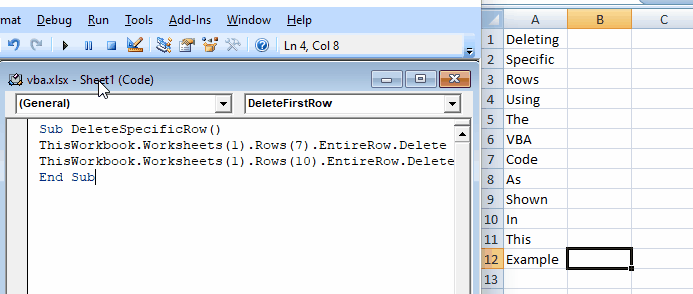The above example shows that when we pass the row number we want to delete, we can easily delete the entire row using the `EntireRow.Delete` method.

## How to Delete Multiple Rows in VBA

We deleted multiple rows using the multiple `EntireRow.Delete` methods in the previous example. But now, we will talk about how we can delete multiple rows with just one method.

We can use the `range` object in VBA to delete multiple rows. The user selects the number of cells meant to be deleted from the Excel sheet first and passes the range as shown below.

``````# VBA
Sub deleteMultipleRow()
Range("A1:C7").EntireRow.Delete
End Sub
``````

Output: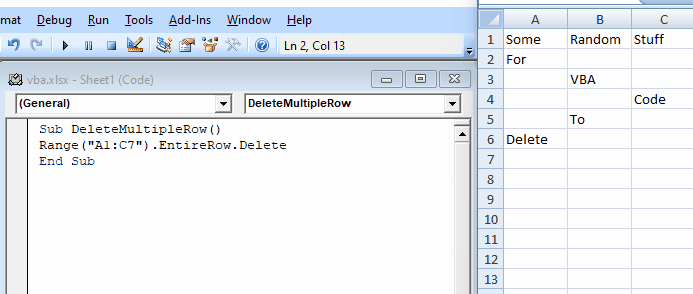The above example shows that using the `Range()` method, we can pass in the range of rows and columns that we want to delete using the `EntireRow.Delete` method.

Multiple rows and columns can directly get removed from the data. We use the VBA code to remove multiple rows (row 2 to row 7) from the data.

``````# VBA
Rows("2:7").Delete
``````

Output: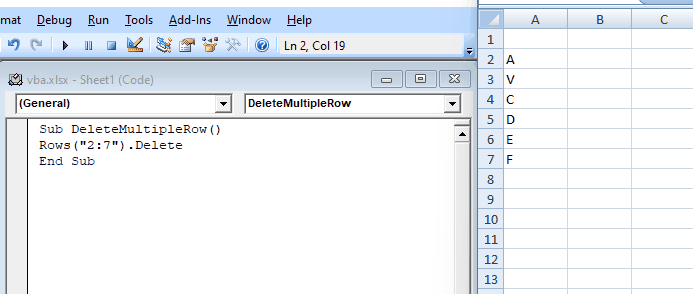If we want to delete a range of columns (from B to C), we can use the following method.

``````# VBA
Columns("B:C").Delete
``````

Output: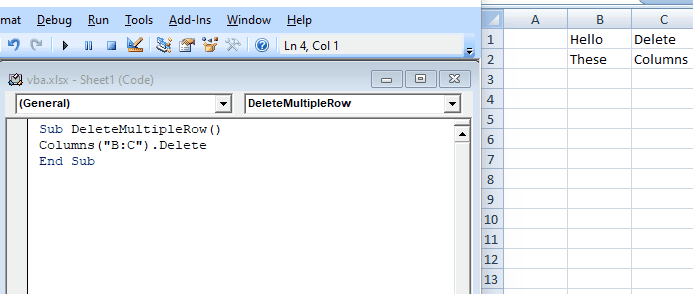## How to Delete Alternative Rows in VBA

Excel sheets are used for many purposes, and it is a common software that is used to save records in the form of Excel sheets. Excel sheets are a basic part of the financial system because most of the data is stored in Excel sheets to find the outcome of that data with formulas.

Some financial Excel sheets contain empty rows on alternative numbers that should be removed to improve the record. VBA helps the user to remove entire alternative rows that are not useful.

As shown below, let’s try to remove the rows with alternative numbers and create a `sub` for this purpose.

``````Sub AlternateRowDel()
rowLen = Selection.Rows.Count
For a = rowLen To 1 Step -2
Selection.Rows(a).EntireRow.Delete
Next a
End Sub
``````

Output: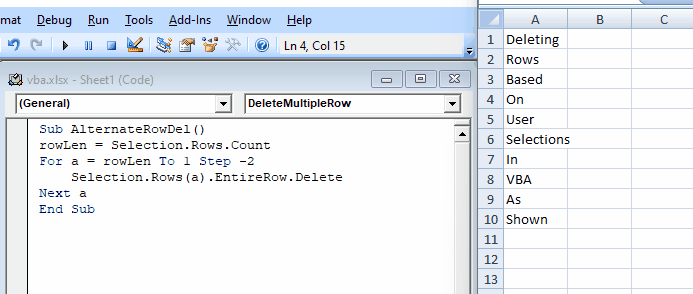As you can see from the above `AlternateRowDel()` sub, we saved the number of rows from the selection to a variable `rowLen` which is the total number of rows to be removed entirely.

Inside our sub, we have a `for` loop that runs with a `Step -2`, which indicates that every second row will be removed. If we want to remove every third row, then write `step -3`, and we can use the same process for any different number of the alternative row that the user wants to remove from the data.

## How to Delete Blank Rows in VBA

When we are working with a large collection of data, there are chances that many or few rows can be empty. We may want to delete them using VBA because it can be time-consuming and hard to find all of them if we start deleting them manually.

Code usually works more accurately than humans when we provide a large data collection. A method, `SpecialCells`, can delete these rows.

We can pass the `xlCellTypeBlanks`, selecting only blank cells. As shown below, let’s go through an example and try to delete all the blank rows using VBA.

``````# VBA
Sub RemoveBlankRows()
Selection.SpecialCells(xlCellTypeBlanks).EntireRow.Delete
End Sub
``````

Output: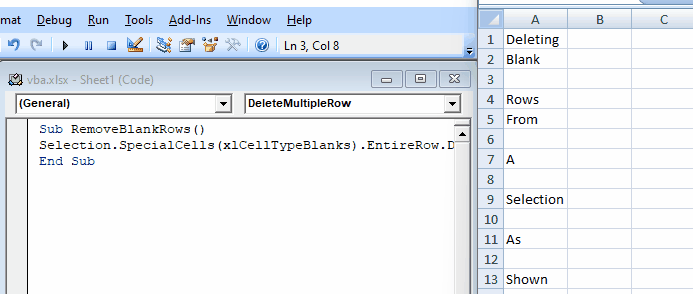The above example shows that we can delete hundreds or thousands of empty rows with one line of code. If we start deleting them manually, it can take hours or days.

## How to Delete Rows With Specific Values in VBA

Sometimes we may come across a situation when we have to delete rows that contain a specific value. And it can be a hard and hectic process to delete these rows manually.

But VBA provides the solution for this problem as well. We can create a custom sub that can iterate through all rows and find that specific value.

If the value is found in any of these rows, we can delete that row using the `EntireRow.Delete` method.

Let’s use this logic in our example, as shown below.

``````# VBA
Sub RemoveWithValue()
For a = Selection.Rows.Count To 1 Step -1
If Cells(a, 1).Value = "Delete" Then
Cells(a, 1).EntireRow.Delete
End If
Next a
End Sub
``````

Output: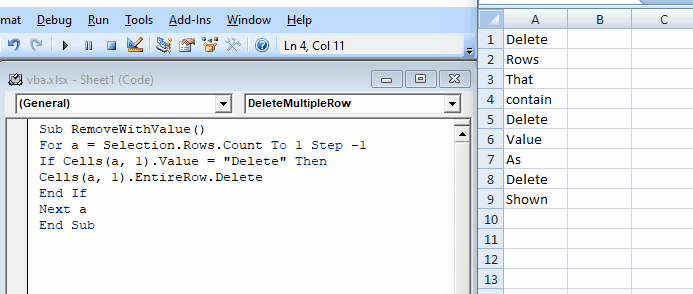The above example shows that this code will iterate through all rows and find the word `delete`. If `delete` was found in any of these rows.

Our code will delete these rows.

## How to Delete Duplicate Rows in VBA

There can be situations where we may have a set of data with many duplicates that can cause problems in some of the results. Delete duplicates can also be hectic if we start doing it manually.

It can also be difficult to remember if a certain entry appeared before.

To make it easier and simpler, we can use another method in VBA, `RemoveDuplicates`. This method takes in a range, finds duplicates, and deletes it.

Let’s use this scenario in our example, as shown below.

``````# VBA
Range("A1:A10").RemoveDuplicates Columns:=1
``````

Output: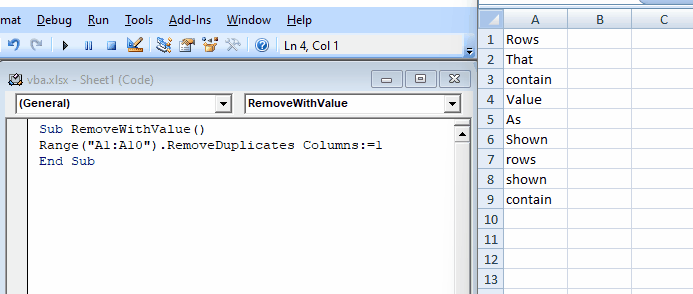The above example shows that we passed the range from the `a1` cell to the `b10` cell. And our code will find any duplicates and delete them.

### How to Use VBA Code in an Excel Sheet

Sometimes people get confused about where they can write a VBA code to run the scripts on their Excel sheets.

Here are a few steps that we can follow to run any of our VBA codes in any Excel sheet we want.

1. First, we need to open the VBA editor. We can easily open the VBA editor by pressing the following buttons.

First, we will hold the ALT key, and then we will press the F11 key.

1. Once the VBA editor is opened, we will go to the `View` option and select `Project Explorer`. We can also get the Project Explorer on the left side of the worksheet when we are entered into the VBA editor mode.
2. Now, we will right-click on the object we want to run the code.
3. Now, we will add a module to our worksheet. We can easily add the module to our worksheet by clicking on the `Insert` and selecting `Module`.
4. We will copy the code we want to paste and paste it. Or, if we want to write the code there, we can do that.
5. We will run the code by keeping the cursor and pressing the F5 key. We can also run the VBA code by clicking on the green triangle present in the VBA toolbar.
Write for us
DelftStack articles are written by software geeks like you. If you also would like to contribute to DelftStack by writing paid articles, you can check the write for us page.

## Related Article - VBA Row

• Find the Last Row and Column in VBA